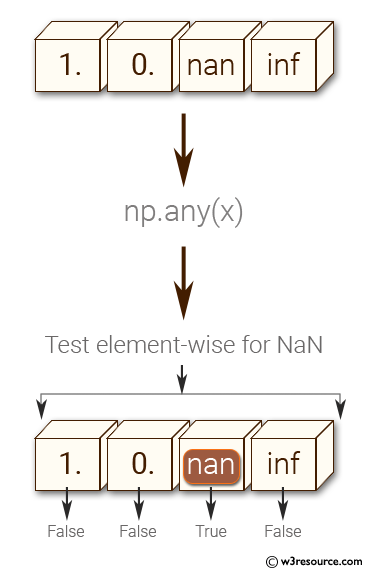﻿ NumPy: Test element-wise for NaN of a given array - w3resource# NumPy: Test element-wise for NaN of a given array

## NumPy: Basic Exercise-7 with Solution

Write a NumPy program to test element-wise for NaN of a given array.

Sample Solution :

Python Code :

``````import numpy as np
a = np.array([1, 0, np.nan, np.inf])
print("Original array")
print(a)
print("Test element-wise for NaN:")
print(np.isnan(a))
``````

Sample Output:

```Original array
[  1.   0.  nan  inf]
Test element-wise for NaN:
[False False  True False]
```

Pictorial Presentation:Python Code Editor:

Have another way to solve this solution? Contribute your code (and comments) through Disqus.

What is the difficulty level of this exercise?

Test your Python skills with w3resource's quiz

﻿

## Python: Tips of the Day

Python: Use Enumerate() In for Loops

```>>> students = ('John', 'Mary',  'Mike')
>>> for i, student in enumerate(students):
...     print(f'Iteration:  {i}, Student: {student}')
...
Iteration: 0, Student: John
Iteration: 1, Student: Mary
Iteration: 2, Student: Mike
>>> for i, student in enumerate(students,  35001):
...      print(f'Student Name: {student}, Student ID #: {i}')
...
Student Name: John, Student ID #: 35001
Student Name: Mary, Student ID #: 35002
Student Name: Mike, Student ID #: 35003
```